Categories

We first look at the program does not have multi-tasking```import time

def sing():
for i in range(5):
print("I like to sing")
time.sleep(1)

def dance():
for i in range(5):
print("I like to dance")
time.sleep(1)

def main():
sing()
dance()
pass

if __name__ == "__main__":
main()```

The result: It took more than ten seconds, can only be performed in sequence, not together / synchronous execution

```I like to sing
I like to sing
I like to sing
I like to sing
I like to sing
I like to dance
I like to dance
I like to dance
I like to dance
I like to dance```

Let us look at the use of multi-threaded programs```import time

def sing():
for i in range(5):
print("I like to sing")
time.sleep(1)

def dance():
for i in range(5):
print("I like to dance")
time.sleep(1)

def main():
t1.start()
t2.start()

if __name__ == '__main__':
main()```

The result: spend a little more than five seconds, execute code synchronization

```I like to sing
I like to dance
I like dancing I like to sing

I like to dance
I like to sing
I like to dance
I like to sing
I like to dance
I like to sing```

Here we can extend some knowledge of how to run a computer program consists of additional multi-tasking?

The operating principle of a single core cpu: round-robin

Single core cpu only run one program at the same time, but you can see a lot of programs running because fast switching single-core cpu, that is to take over a program running very short period of time such as .00001 seconds, you run a program change , and so forth, that is, at the same time you see the execution of multiple programs. This is one way to achieve multi-tasking operating system, but in fact is a pseudo multi-tasking.

Round-robin philosophy is that as long as I switched fast enough, you see what I do more than one thing at the same time, this is the scheduling algorithms operating system. Operating system as well as priority scheduling, such as listening to music to be continued.

If multicore cpu to run multiple tasks at the same time, we call parallel, true multi-tasking; the number of tasks is less than the number of cpu;

If the switch is a single core cpu to run multiple tasks, we call it concurrent, multi-tasking is false. The number of tasks than the number of CPU;

But because the daily, the number of tasks usually more than the number of cpu core, so we are talking about multi-tasking are generally concurrent, or false multi-task;

Threading.Thread create instances of the class object and pass a function reference;

Call start method instance of an object, create a child thread.

If you do not know how to use multithreading? It does not matter, look at the following chart to know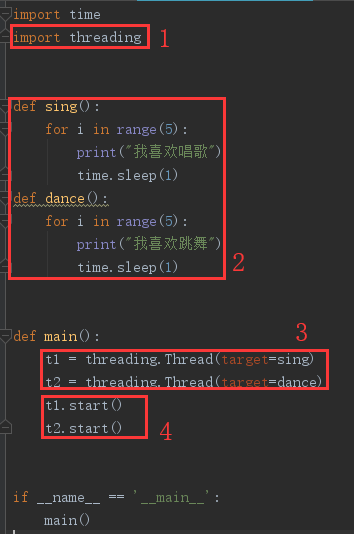code show as below:```import time

def sing():
for i in range(5):
print("I like to sing")
time.sleep(1)

def dance():
for i in range(5):
print("I like to dance")
time.sleep(1)

def main():
t1.start()
t2.start()

if __name__ == '__main__':
main()```

note:

() Function call represents the function name

Function name indicates the use of a reference function, tells the function in which;

```def main():
t1.start()
t2.start()```

Each function will be performed when there is a thread, the main thread we call; when we perform a

`t1 = threading.Thread(target=sing)`

When, you create an instance of an object represents a Thread class t1, and pass references to a Thread class sing function of t1. Similarly, t2 is also true; when we execute to

`t1.start()`

The main thread to wait for the end of the sub-thread execution reason: child thread in the implementation process will call resources and produce some variables, when the child thread executing the main thread you want these useless resources and garbage cleanup.

We can use the following code to get all threads in the current program;

`threading.enumerate()`

On the use of enumerate, you can view a blog on my python functions of the built-in function enumerate, but represented here is to get all threads in the current program, you can not see;

Because after the thread creation, thread execution order is uncertain, if we want the method to perform a thread, you can use the time.sleep. code show as below```import time

def sing():
for i in range(5):
print("-----sing----%d" % i)

def dance():
for i in range(5):
print("-----dance----%d" % i)

def main():
t1.start()
time.sleep(1)
print("sing")

t2.start()
time.sleep(1)
print("dance")

if __name__ == '__main__':
main()```

operation result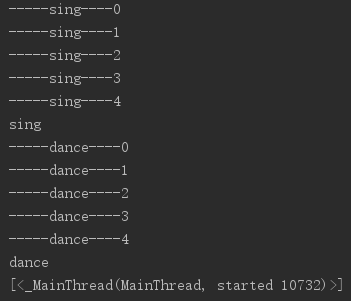We can see, sing threads have to run, but only one thread at this time to see the main thread, this is because when the child thread execution finished before viewing thread to execute the code.

To cycle through the currently running thread count

We can many times, the main thread to see infinite loop by letting the child thread delay execution of the current number of threads (appropriate delay), you can see the number of the currently running thread, when the thread number 1 or less, use the break ends the main thread.

code show as below```import time

def sing():
for i in range(5):
print("-----sing--%d--" % i)
time.sleep(1)

def dance():
for i in range(5):
print("-----dance--%d--" % i)
time.sleep(1)

def main():
t1.start()
t2.start()
while True:
#print ( "The current number of threads running:% s"% t_len)
if t_len <= 1:
break
time.sleep(1)

if __name__ == '__main__':
main()```

To cycle through the currently running thread count

operation result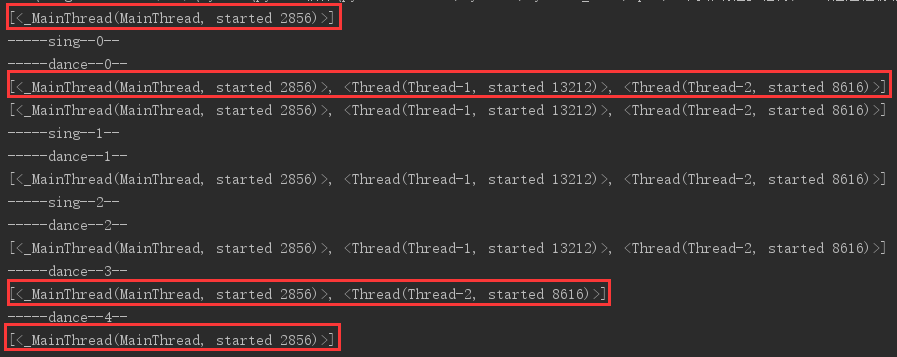We can see, the beginning of time is only one main thread, when the child thread start, there are three threads, sing at the end of sub-thread, leaving only two threads, and after the end of the dance, there is only one main thread.

Verify the execution time of the child thread

In order to verify the execution time of the child thread, we can run the code in interactive python, a function called in when the child thread execution which represents a sub-thread execution and when;

Verification results are as follows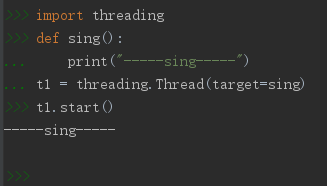After the execution of this, we can determine the child thread execution start is to call the object in the example of the thread () method.

Authentication Code

```import threading
def sing():
print("-----sing-----")
t1.start()
-----sing-----```

Verify the creation time of the child thread

Verification principle: we can number each time period to determine the child thread was created by computing the number of threads

Authentication Code```import time

def sing():
for i in range(5):
print("----sing----")
time.sleep(1)

def main():
print("The number of threads before creating an instance of an object:", len(threading.enumerate()))
print("Before the number of threads / start following method creates an instance of an object:", len(threading.enumerate()))
t1.start()

if __name__ == '__main__':
main()```

Verify the creation time of the child thread

Validation results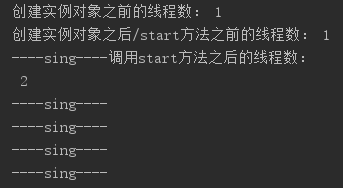The number of threads can be observed before calling the start method has always been a main thread, so we can determine the time for a thread is created in the instance of an object method calls start after;

Combined with the front, we can conclude that create time and execution time the child thread is created out of the Thread instance object after calling the start method, and the end of time the child thread is executed after the function call is completed.

To create a process through inheritance Thread class

We are in front of the child thread by calling a function, then when the function too, want those functions packaged as a class, we can not call a class by sub-thread of it?

The second method steps of creating a thread

Define a class, the class which inherits threading.Thread class, which defines a run method;

Then create an instance of an object class;

Call an instance object’s start method, you create a thread.

If you create a thread through the class inheritance is when a Thread class to create, you must define the run method in which, when you start method is called, it will automatically call the run method is to run the next method of executing code inside thread .

The sample code to create a process through inheritance Thread class```import time

def run(self):
print("---run---")
for i in range(3):
msg = "I'm% s, i --->% s" % (self.name, str(i))  #Self.name is saved in the name of the current thread
print(msg)
time.sleep(1)

def main():
t1.start()

if __name__ == '__main__':
main()```

To create a process through inheritance Thread class

operation result

```---run---I am a Thread-1,i--->0

Knowledge Point

This method is applicable to a thread inside to do more complicated, to be packaged into several functions to do, then we will package it into a class.

Several other functions defined in the class, you can call these functions run inside.

Which method is better when you create a thread? Which is simple to use which.

note:

An instance of an object can only create a thread; time to create a process through inheritance Thread class, the class does not automatically call a function in addition to other functions run, if you want to call other numbers, you can use self.xxx in the run method () to call.

Modify global variables in a function, if it is digital and other immutable types, then use the global statement can be modified, such as if it is a list of variable types, you can not declare, and other direct append to the list of content to be modified, but, if not to modify the contents of the list, but point to a new list, you need to use the global statement;

In a global variable, if the data is referenced to modify, you need to use global, if it is modified (for a direct reference address) references to global variables, then you need to use global, at the same time, we should also pay attention to the global variable or variables are immutable type, such as a digital, immutable, can only be modified by modifying the variable global variable references, it is necessary to global;

Verify multiple threads share global variables

Verification principle:

`定义一个全局变量，在函数1中加1，在函数2中查看，让线程控制的函数1先执行，如果线程函数2的查看结果和函数1的查看结果一样，那么就证明多线程之间共享全局变量。`

Code verification```import time

g_num = 100

def sing():
global g_num
g_num += 1
print("--- Sing in g_num:% d ---" % g_num)
time.sleep(1)

def dance():
print("--- Dance in g_num:% d ---" % g_num)
time.sleep(1)

def main():
t1.start()
t2.start()
print("--- the main thread of g_num:% d ---" % g_num)

if __name__ == '__main__':
main()```

Sharing global variables between multiple threads verification

operation result

```---sing中的g_num: 101---
---dance中的g_num: 101---
---主线程中的g_num: 101---```

As we can see the code, we share global variables between multiple threads.

We can share global variables between multiple threads to be understood as: a house, there are several people, a person is a thread, everyone has things their own private resources, but in the big house there, there are also some things, such as He said the only fountain of water, have a drink half, scored a man to pick water, only half of this water dispenser inside who is the global variable.

Pass parameters to the child thread syntax is as follows

```g_nums = [11, 22]

Parameter passing to the sub-thread Sample Code```import time

def sing(temp):
temp.append(33)
print("--- sing in g_nums:% s ---" % str(temp))
time.sleep(1)

def dance(temp):
print("--- dance in g_nums:% s ---" % str(temp))
time.sleep(1)

g_nums = [11, 22]

def main():
t1.start()
time.sleep(1)
t2.start()
time.sleep(1)
print("--- the main thread of g_nums:% s ---" % str(g_nums))

if __name__ == '__main__':
main()```

Pass parameters to the child thread

operation result

```---sing中的g_nums: [11, 22, 33]---
---dance中的g_nums: [11, 22, 33]---
---主线程中的g_nums: [11, 22, 33]---```

Sharing between multiple threads: competition for resources

Shared global variables exist resource contention problems, two threads will use or modify the existing problems, a modification that uses not exist; pass parameters when 100 might not be a problem, because the number is small, the probability of dots; However, parameter passing 1000000 when digital becomes large, the probability increases;

num + = 1 can be divided into three, the value of the num acquired, a value plus 1, for reassignment num; 1 is possible when performing the thread 12, is intended to implement the three, cpu will resources to thread 2 , and the thread 2 the same way, then the implementation of the first three threads 1, so the thread 1 +1, global variables to store 1; 2 + 1 thread turn, storing global variables 1; the question arises, would add two should be 2, but still a global variable.

Competition for resources code examples```import time

g_num = 0

global g_num
for i in range(count):
g_num += 1

global g_num
for i in range(count):
g_num += 1

def main():

t1.start()
t2.start()
time.sleep(3)
print("the g_num of main:", g_num)

if __name__ == '__main__':
main()```

Shared variable resource competition

operation result

```the g_num of add1: 1096322
the g_num of main: 1294601```

Mutex to solve the problem of resource contention

Atomic operations: either do or done;

Mutex: a person to do something, other people are not allowed to do this, you must wait until the person in front to be done about it, then to do the examples attractions toilet.

Mutex grammar

```#Create a lock:
#Lock:
mutex.acquire()
#Unlock:
mutex.release()```

Use a mutex to solve the problem of resource contention```import time

g_num = 0

global g_num
for i in range(num):
mutex.acquire()
g_num += 1
mutex.release()

global g_num
for i in range(num):
mutex.acquire()
g_num += 1
mutex.release()

def main():
t1.start()
t2.start()

time.sleep(2)
print("the g_num of main:", g_num)

if __name__ == '__main__':
main()```

Use a mutex to solve the problem of resource contention

operation result

```the g_num of add2: 1901141
the g_num of main: 2000000```

As can be seen, the use of mutex can resolve resource contention problems.

In particular, the use of multiple mutex mutex time, especially prone to deadlock, what you waiting for my resources, I’m waiting for your resources;

This chapter summarizes

From the beginning to the end of the execution, there has been a main thread

If the main thread to die first, then the child thread running will die.

Child thread is created at the beginning when calling t.start (), instead of creating an instance of the Thread object.

Child thread is started when t.start () call;

Time of death is in the child thread after thread of the call function execution is completed;

After the thread is created, a thread of execution order is uncertain;

If you want to make a thread to execute, time.sleep method can be used.

Threading.Thread create instances of the class object and pass a function reference;

Call start method instance of an object, create a child thread.

To create a process through inheritance Thread class

Define a class, the class which inherits threading.Thread class, which defines a run method;

Then create an instance of an object class;

Call an instance object’s start method, you create a thread.

Creating multiple threads can be understood in preparation for the creation of threads;

start () is directly create and run the thread when you’re ready;

After the main thread to wait for the child thread in the end is to clean up garbage in the child thread that may arise;